# Geometrical Optics Questions and Answers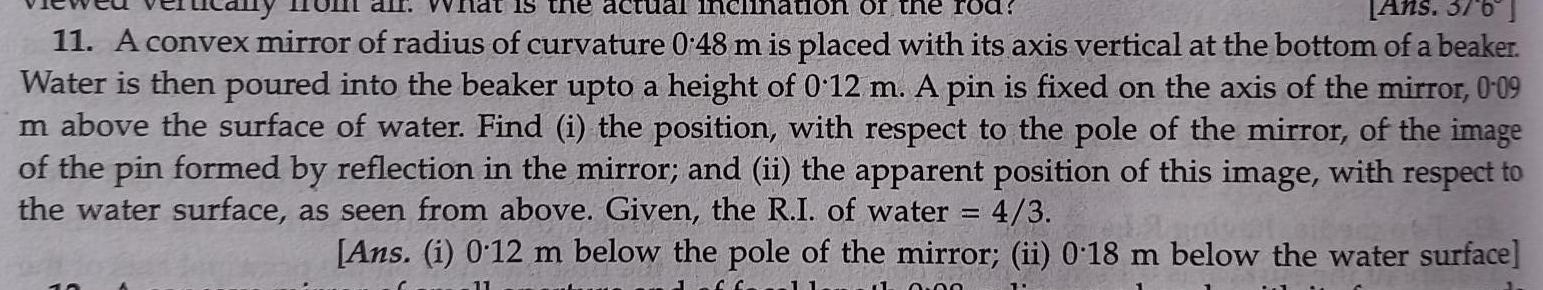Physics
Geometrical Optics
is the actual inclination of the rod Ans 376 11 A convex mirror of radius of curvature 0 48 m is placed with its axis vertical at the bottom of a beaker Water is then poured into the beaker upto a height of 0 12 m A pin is fixed on the axis of the mirror 0 09 m above the surface of water Find i the position with respect to the pole of the mirror of the image of the pin formed by reflection in the mirror and ii the apparent position of this image with respect to the water surface as seen from above Given the R I of water 4 3 Ans i 0 12 m below the pole of the mirror ii 0 18 m below the water surface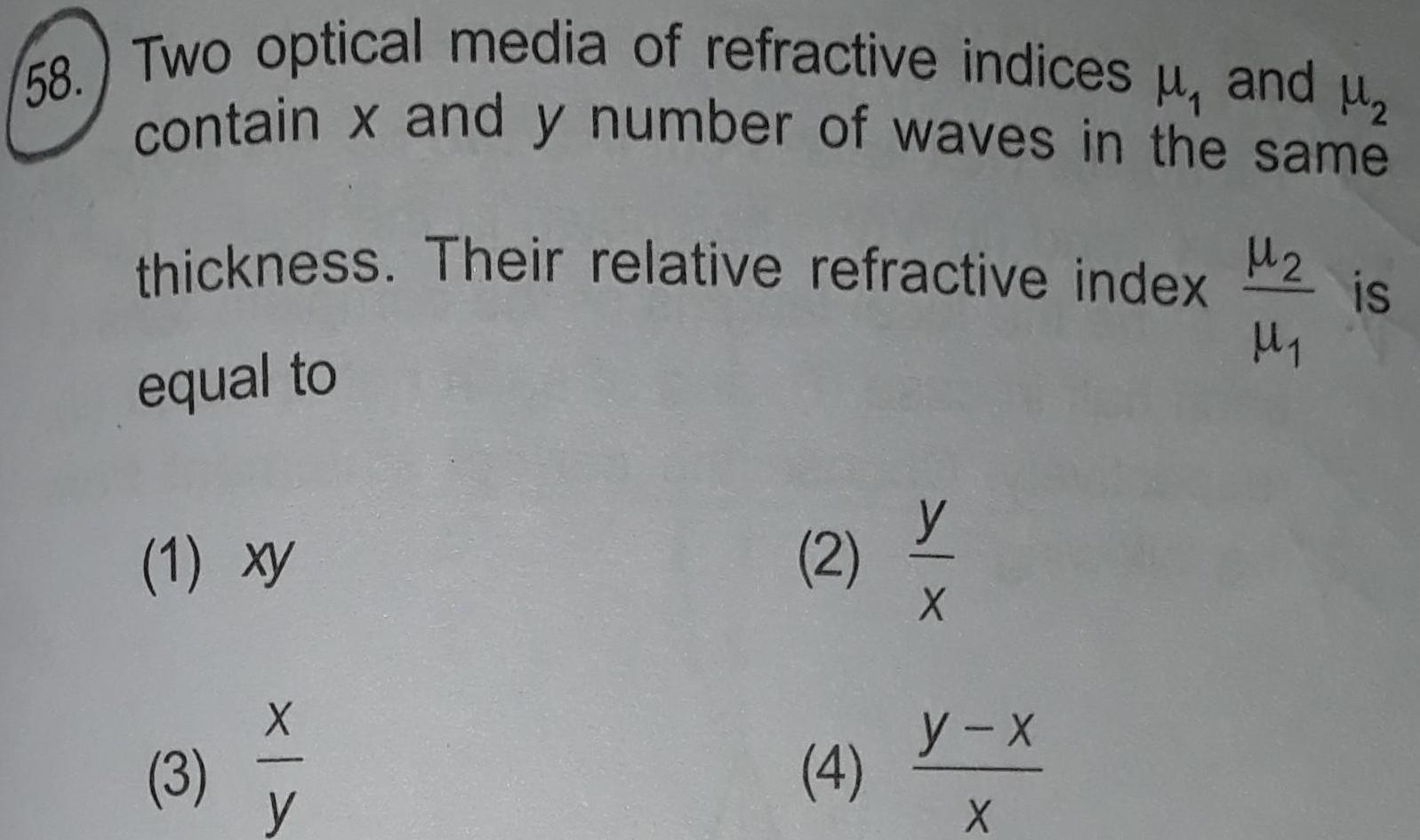Physics
Geometrical Optics
58 Two optical media of refractive indices and contain x and y number of waves in the same thickness Their relative refractive index equal to 1 xy 3 X y 2 4 y X y x X M2 is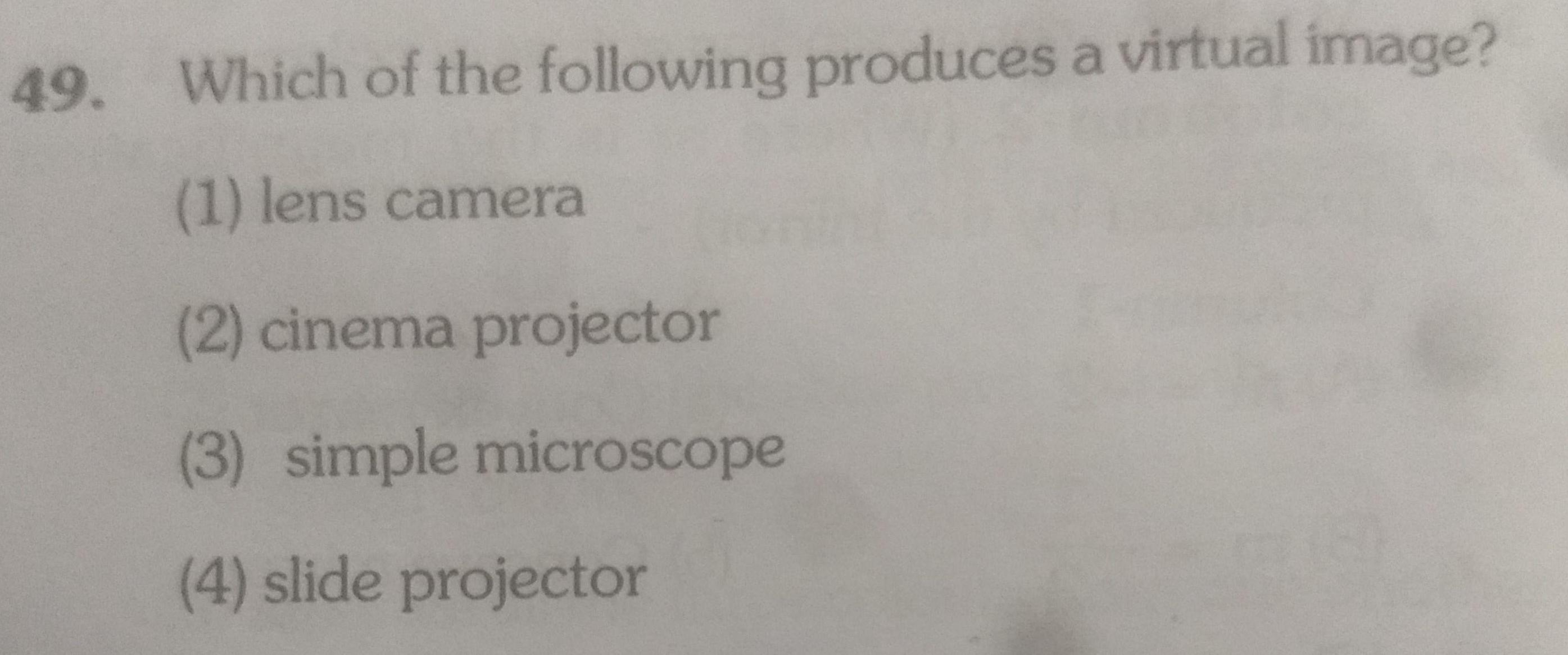Physics
Geometrical Optics
49 Which of the following produces a virtual image 1 lens camera 2 cinema projector 3 simple microscope 4 slide projector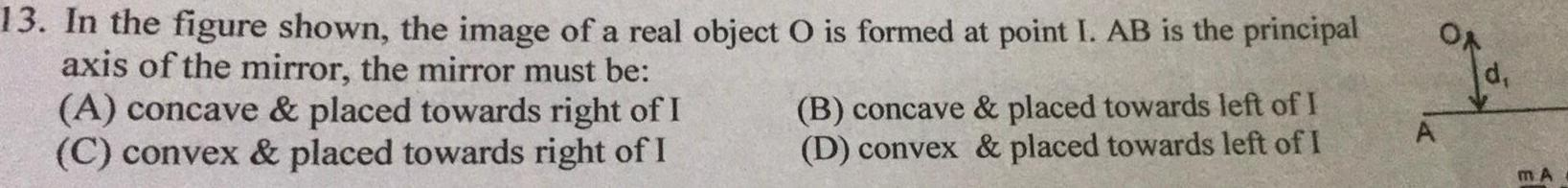Physics
Geometrical Optics
13 In the figure shown the image of a real object O is formed at point I AB is the principal axis of the mirror the mirror must be A concave placed towards right of I C convex placed towards right of I B concave placed towards left of I D convex placed towards left of I m A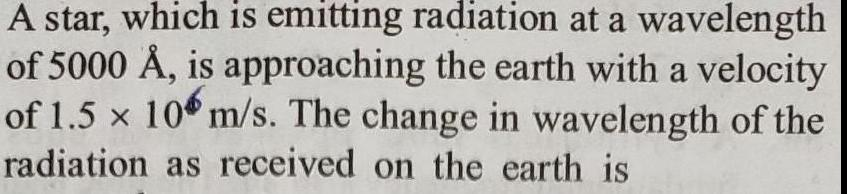Physics
Geometrical Optics
A star which is emitting radiation at a wavelength of 5000 is approaching the earth with a velocity of 1 5 x 10 m s The change in wavelength of the radiation as received on the earth is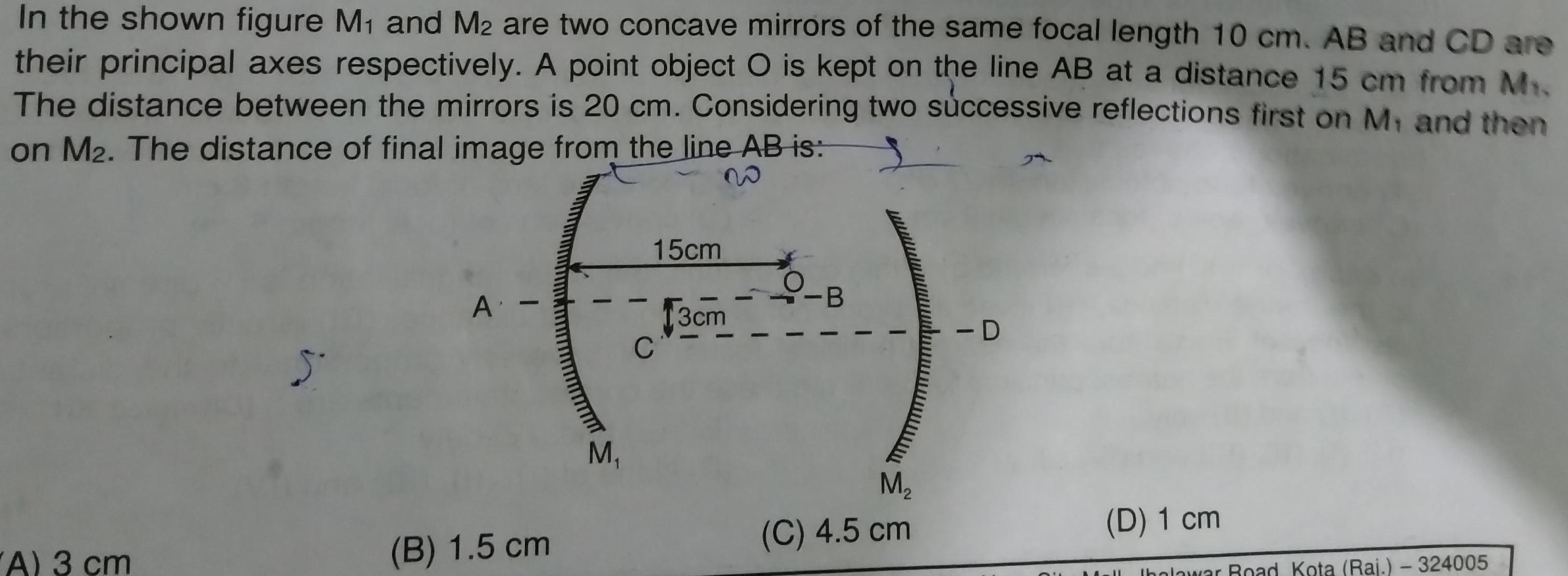Physics
Geometrical Optics
In the shown figure M and M2 are two concave mirrors of the same focal length 10 cm AB and CD are their principal axes respectively A point object O is kept on the line AB at a distance 15 cm from M The distance between the mirrors is 20 cm Considering two successive reflections first on M and then on M2 The distance of final image from the line AB is S A 3 cm 5 A B 1 5 cm M 15cm C 13cm M C 4 5 cm D D 1 cm Iholawar Road Kota Raj 324005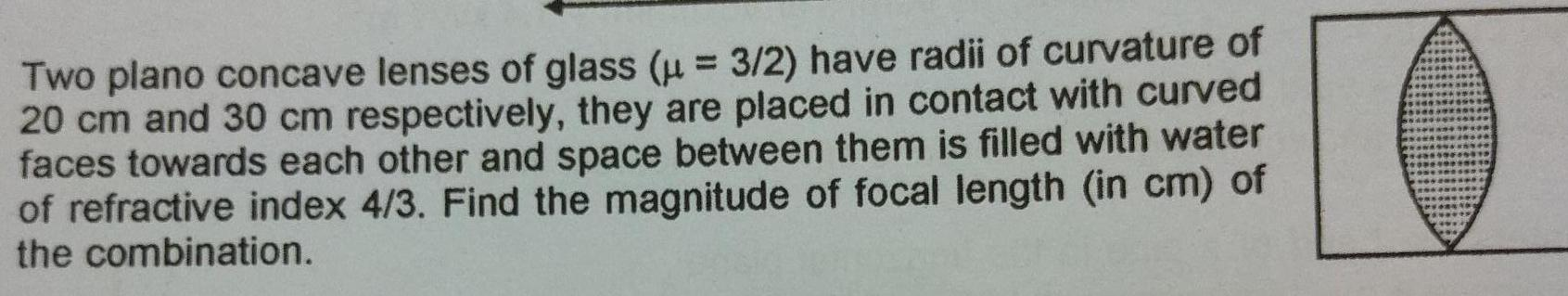Physics
Geometrical Optics
Two plano concave lenses of glass 3 2 have radii of curvature of 20 cm and 30 cm respectively they are placed in contact with curved faces towards each other and space between them is filled with water of refractive index 4 3 Find the magnitude of focal length in cm of the combination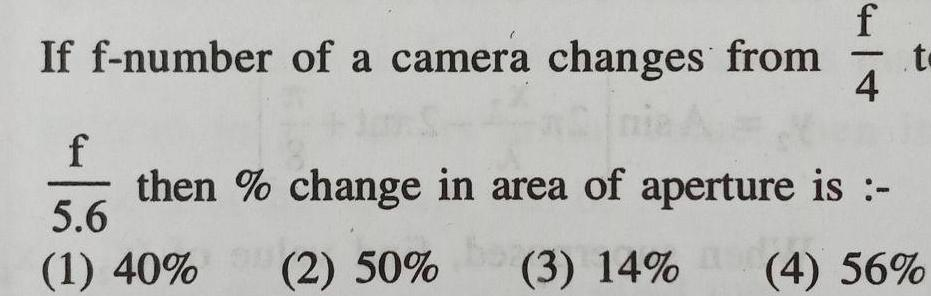Physics
Geometrical Optics
If f number of a camera changes from f 5 6 1 40 f 4 t then change in area of aperture is 2 50 bo 3 14 4 56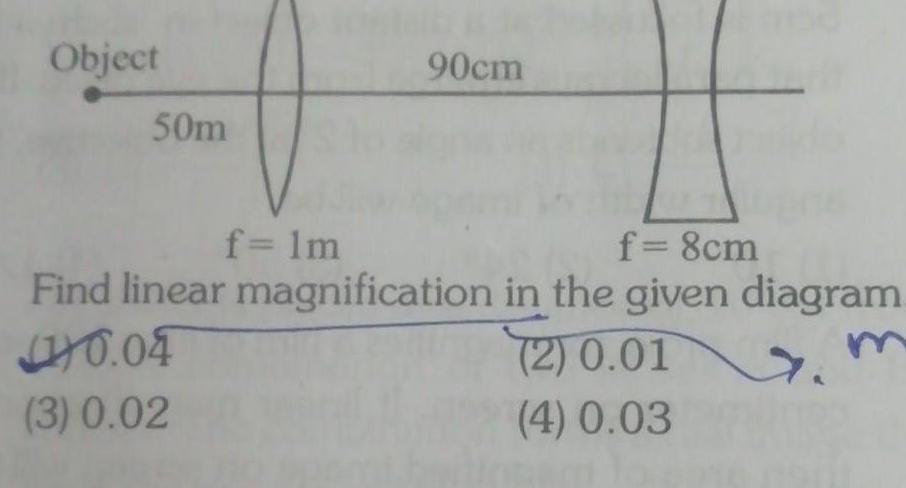Physics
Geometrical Optics
Object 50m 90cm f 1m f 8cm Find linear magnification in the given diagram 2 0 01 m 4 0 03 0 04 3 0 02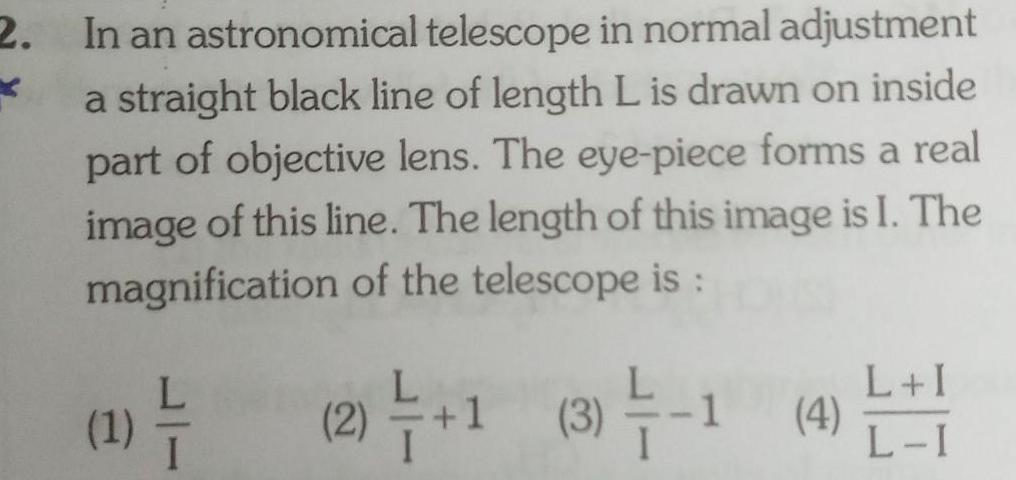Physics
Geometrical Optics
2 In an astronomical telescope in normal adjustment a straight black line of length L is drawn on inside part of objective lens The eye piece forms a real image of this line The length of this image is I The magnification of the telescope is 1 2 1 3 1 4 L I L I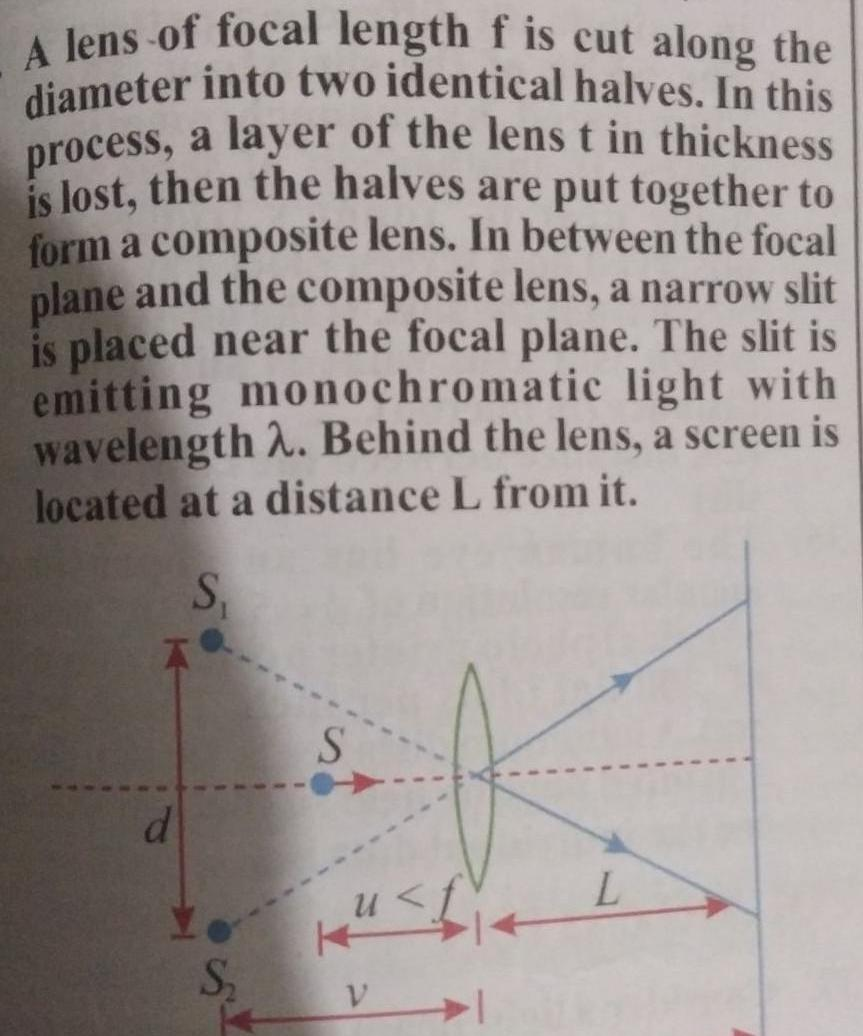Physics
Geometrical Optics
A lens of focal length f is cut along the diameter into two identical halves In this process a layer of the lens t in thickness is lost then the halves are put together to form a composite lens In between the focal plane and the composite lens a narrow slit is placed near the focal plane The slit is emitting monochromatic light with wavelength 2 Behind the lens a screen is located at a distance L from it S d S S V 114 L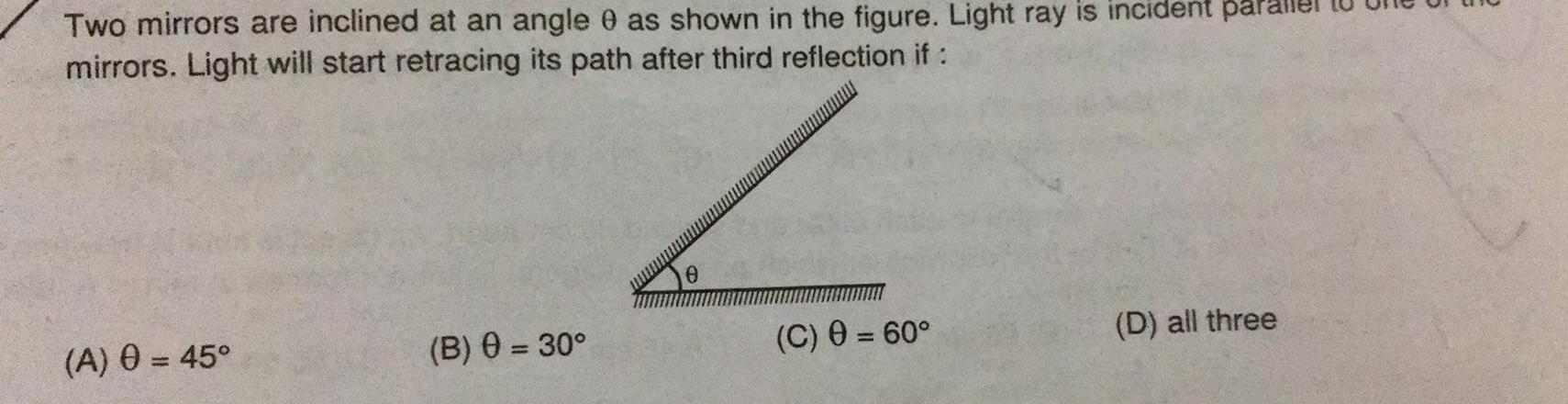Physics
Geometrical Optics
Two mirrors are inclined at an angle 0 as shown in the figure Light ray is incident pal mirrors Light will start retracing its path after third reflection if A 0 45 B 0 30 0 C 0 60 D all three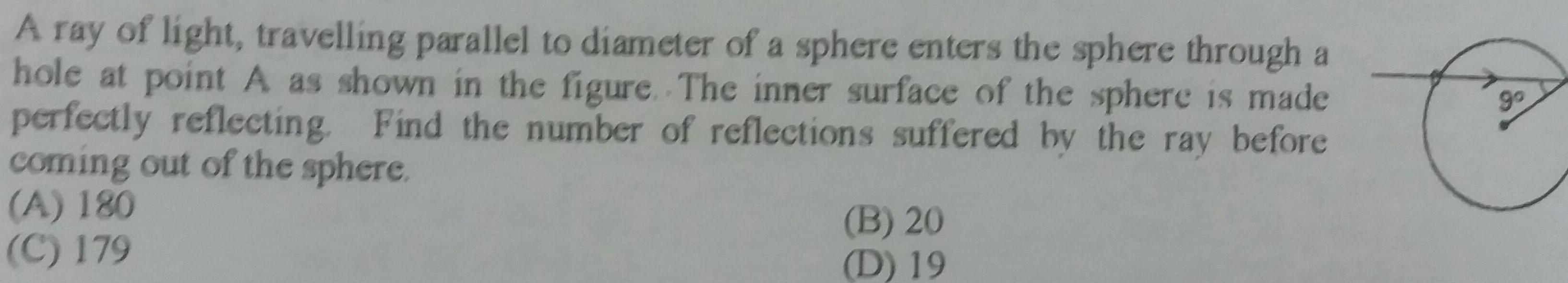Physics
Geometrical Optics
A ray of light travelling parallel to diameter of a sphere enters the sphere through a hole at point A as shown in the figure The inner surface of the sphere is made perfectly reflecting Find the number of reflections suffered by the ray before coming out of the sphere A 180 C 179 B 20 D 19 90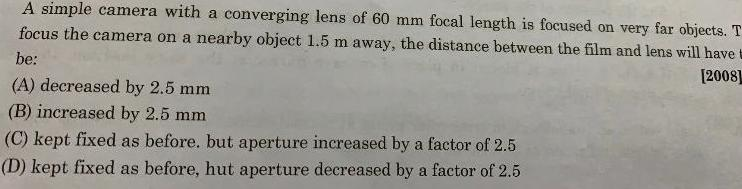Physics
Geometrical Optics
A simple camera with a converging lens of 60 mm focal length is focused on very far objects T focus the camera on a nearby object 1 5 m away the distance between the film and lens will have t be 2008 A decreased by 2 5 mm B increased by 2 5 mm C kept fixed as before but aperture increased by a factor of 2 5 D kept fixed as before hut aperture decreased by a factor of 2 5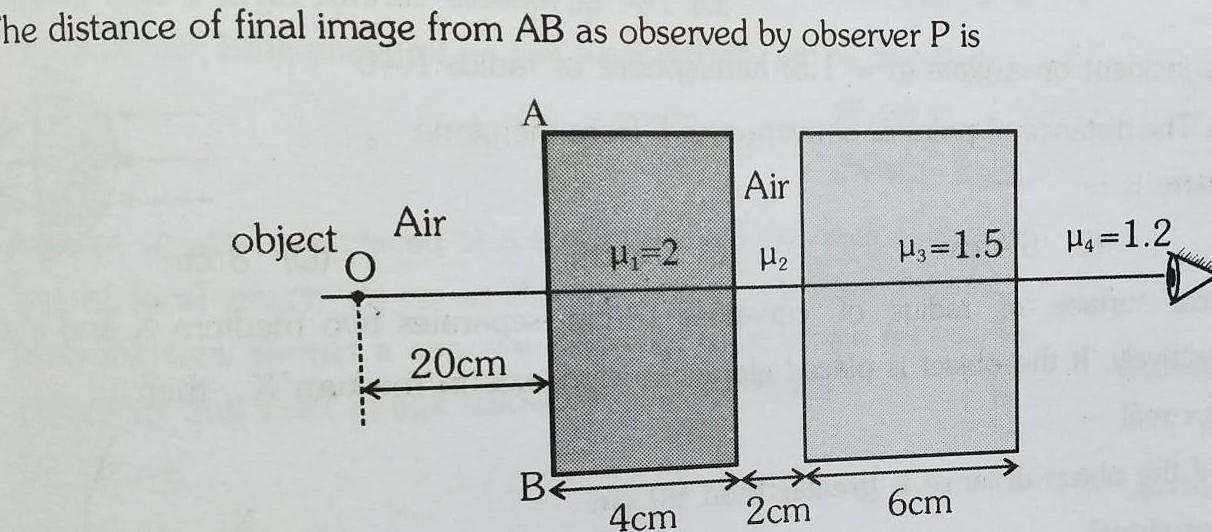Physics
Geometrical Optics
The distance of final image from AB as observed by observer P is A object Air O 20cm BE H 2 Air H 4cm 2cm H3 1 5 6cm P 1 2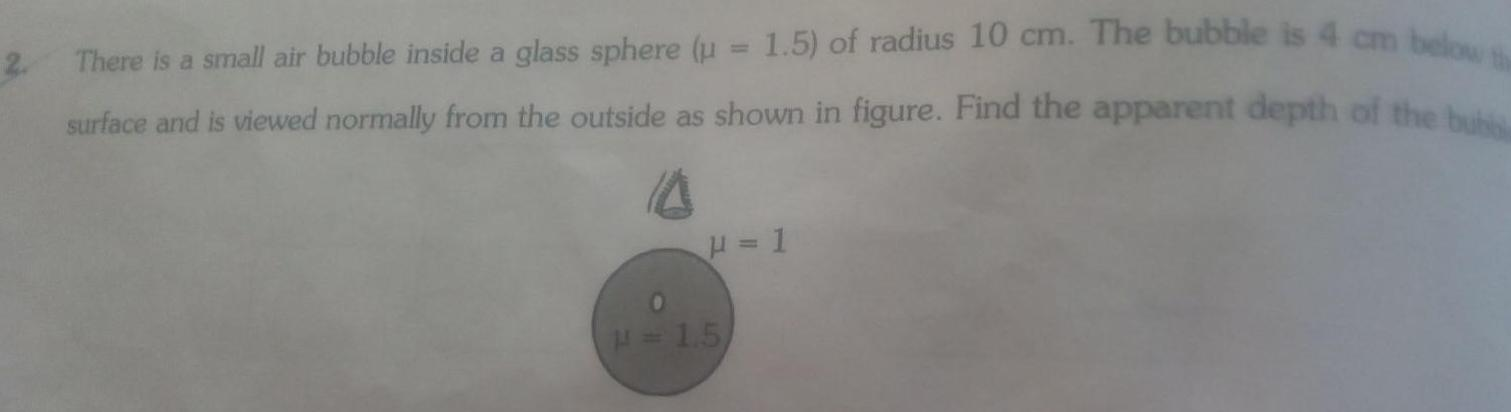Physics
Geometrical Optics
2 There is a small air bubble inside a glass sphere u 1 5 of radius 10 cm The bubble is 4 cm below surface and is viewed normally from the outside as shown in figure Find the apparent depth of the bub H 1 0 H 1 5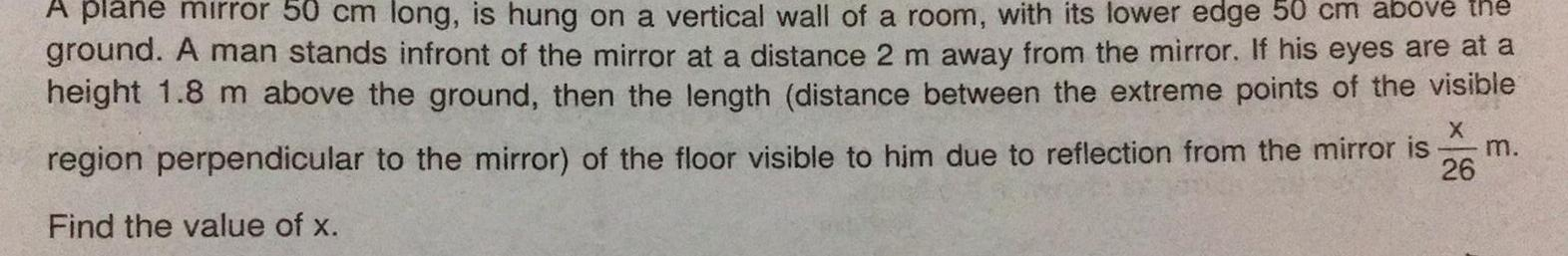Physics
Geometrical Optics
A plane mirror 50 cm long is hung on a vertical wall of a room with its lower edge 50 cm above the ground A man stands infront of the mirror at a distance 2 m away from the mirror If his eyes are at a height 1 8 m above the ground then the length distance between the extreme points of the visible X 26 region perpendicular to the mirror of the floor visible to him due to reflection from the mirror is Find the value of x m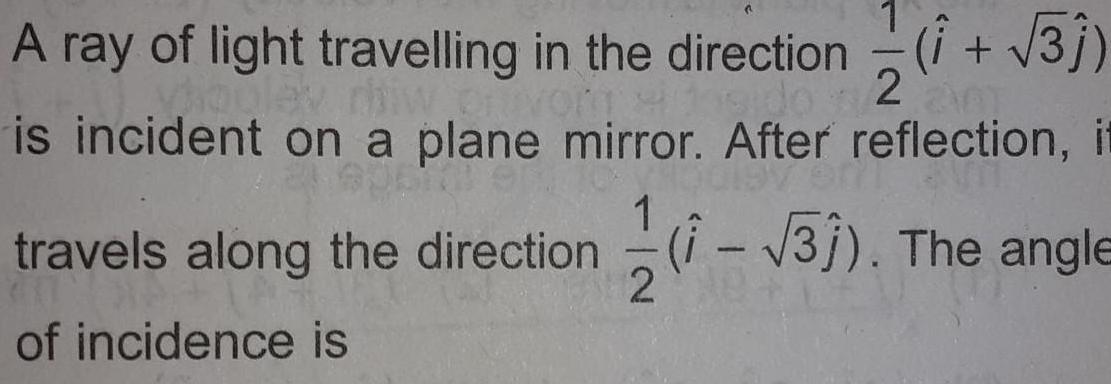Physics
Geometrical Optics
A ray of light travelling in the direction i 31 onvon M sido is incident on a plane mirror After reflection it travels along the direction 1 31 The angle of incidence is 1 2 2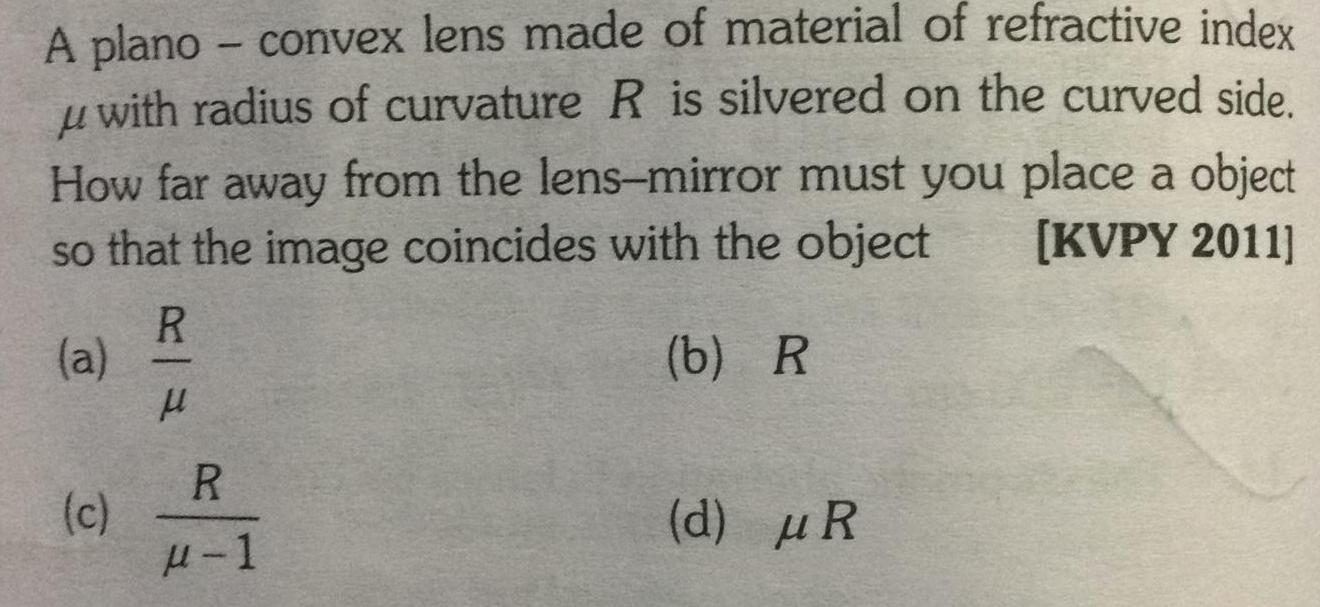Physics
Geometrical Optics
A plano convex lens made of material of refractive index with radius of curvature R is silvered on the curved side How far away from the lens mirror must you place a object so that the image coincides with the object KVPY 2011 R a b R c R 1 d R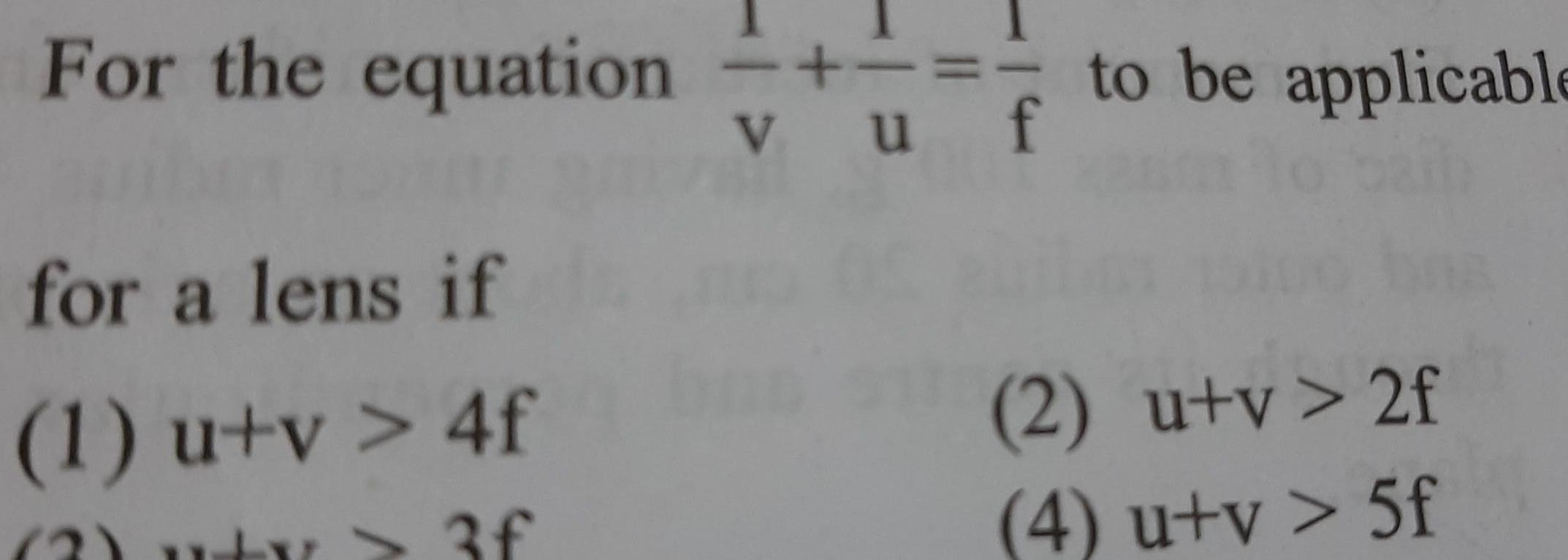Physics
Geometrical Optics
For the equation vuf for a lens if 1 u v 4f wty 3f to be applicable 2 u v 2f 4 u v 5f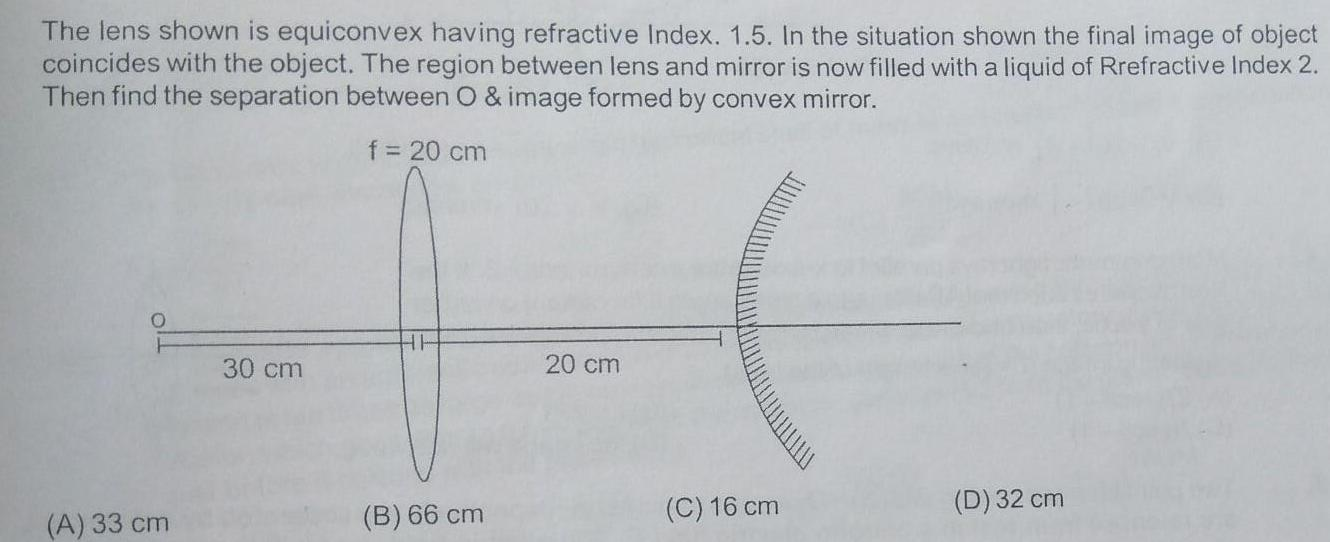Physics
Geometrical Optics
The lens shown is equiconvex having refractive Index 1 5 In the situation shown the final image of object coincides with the object The region between lens and mirror is now filled with a liquid of Rrefractive Index 2 Then find the separation between O image formed by convex mirror f 20 cm A 33 cm 30 cm HH B 66 cm 20 cm C 16 cm D 32 cm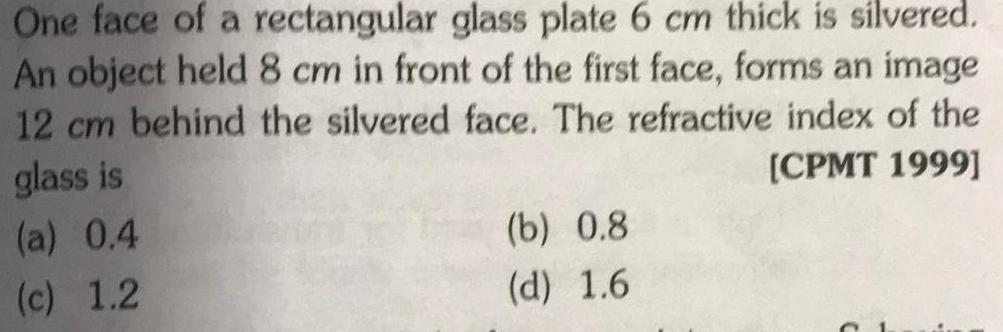Physics
Geometrical Optics
One face of a rectangular glass plate 6 cm thick is silvered An object held 8 cm in front of the first face forms an image 12 cm behind the silvered face The refractive index of the glass is CPMT 1999 a 0 4 c 1 2 b 0 8 d 1 6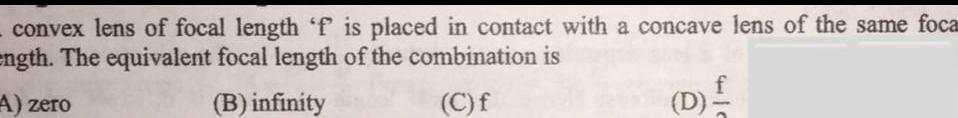Physics
Geometrical Optics
convex lens of focal length f is placed in contact with a concave lens of the same foca ength The equivalent focal length of the combination is A zero B infinity C f D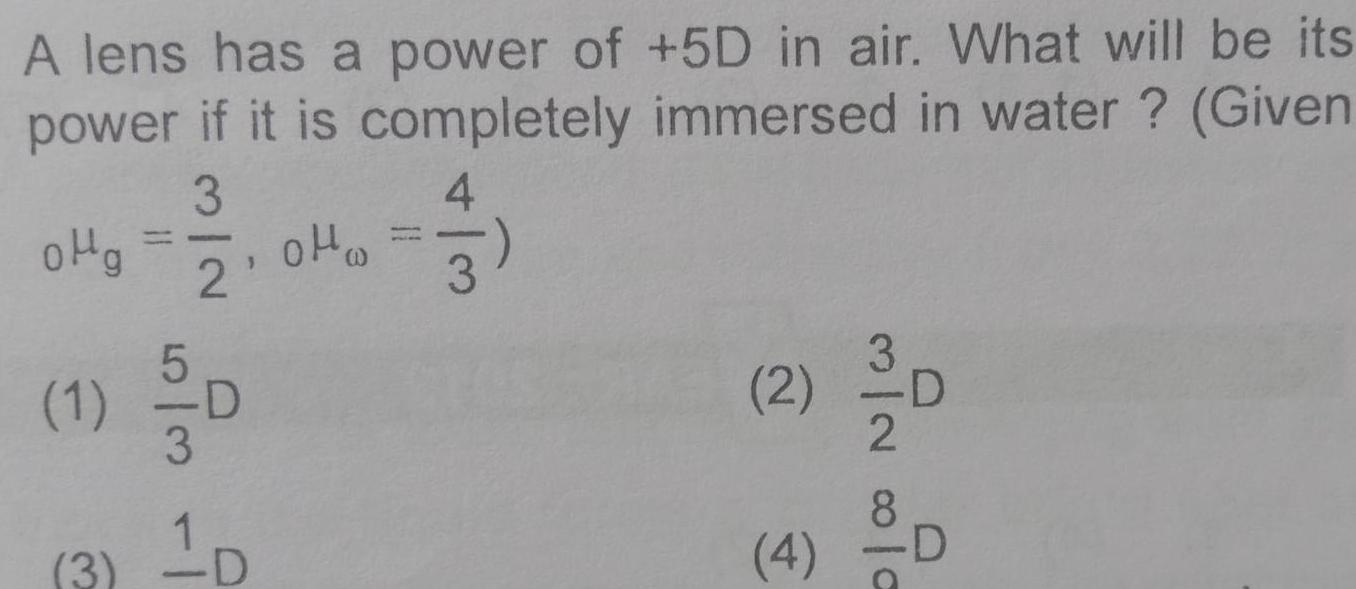Physics
Geometrical Optics
A lens has a power of 5D in air What will be its power if it is completely immersed in water Given 3 oHg 1 4 2 0H 3 2 5 3 3 1D 2 4 MIN OTO D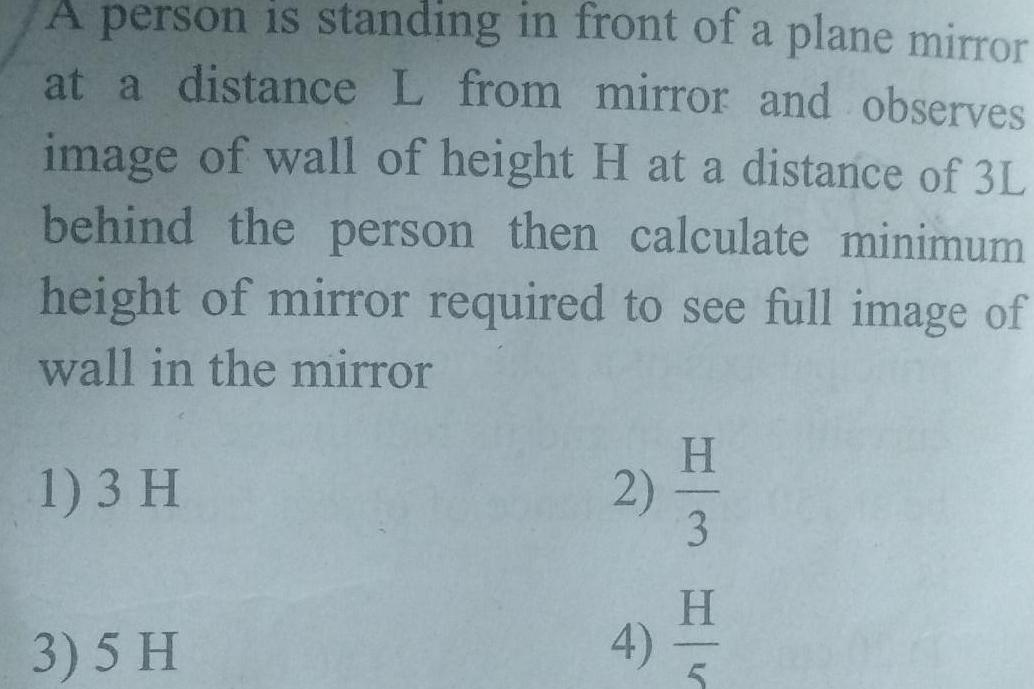Physics
Geometrical Optics
A person is standing in front of a plane mirror at a distance L from mirror and observes image of wall of height H at a distance of 3L behind the person then calculate minimum height of mirror required to see full image of wall in the mirror 1 3 H 3 5 H 2 4 H 3 H 5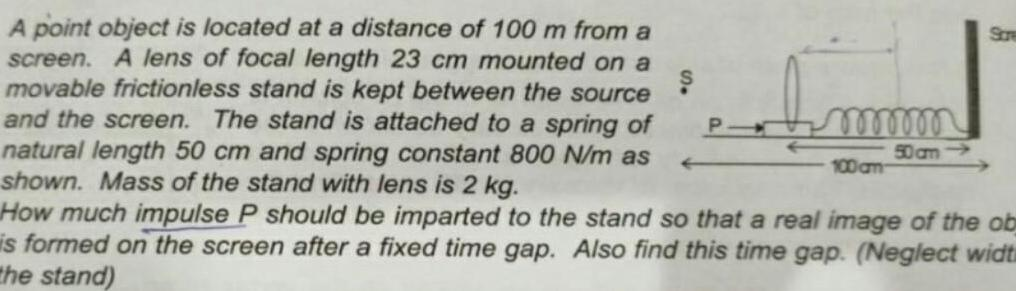Physics
Geometrical Optics
A point object is located at a distance of 100 m from a screen A lens of focal length 23 cm mounted on a movable frictionless stand is kept between the source and the screen The stand is attached to a spring of natural length 50 cm and spring constant 800 N m as shown Mass of the stand with lens is 2 kg How much impulse P should be imparted to the stand so that a real image of the ob is formed on the screen after a fixed time gap Also find this time gap Neglect widt the stand P ooooooo 50 cm 100 cm Stre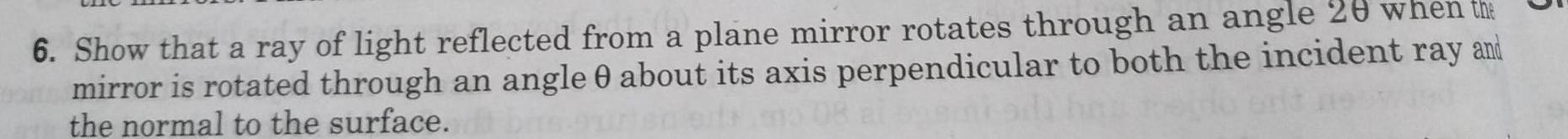Physics
Geometrical Optics
the 6 Show that a ray of light reflected from a plane mirror rotates through an angle 20 when mirror is rotated through an angle 0 about its axis perpendicular to both the incident ray and 08 al enem the normal to the surface 5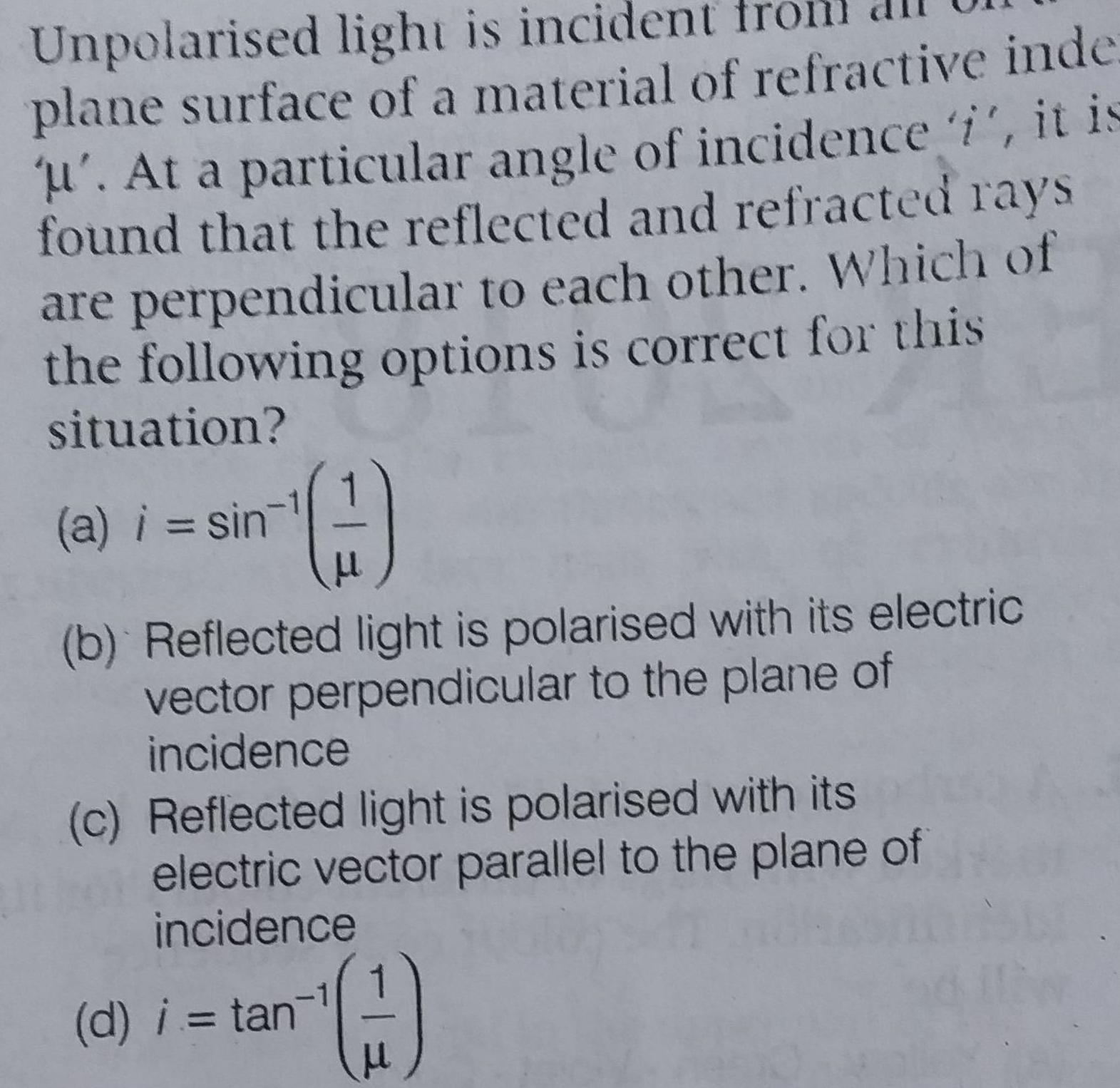Physics
Geometrical Optics
Unpolarised light is incident fro plane surface of a material of refractive inde u At a particular angle of incidence i it is found that the reflected and refracted rays are perpendicular to each other Which of the following options is correct for this situation a i sin b Reflected light is polarised with its electric vector perpendicular to the plane of incidence c Reflected light is polarised with its electric vector parallel to the plane of incidence d i tan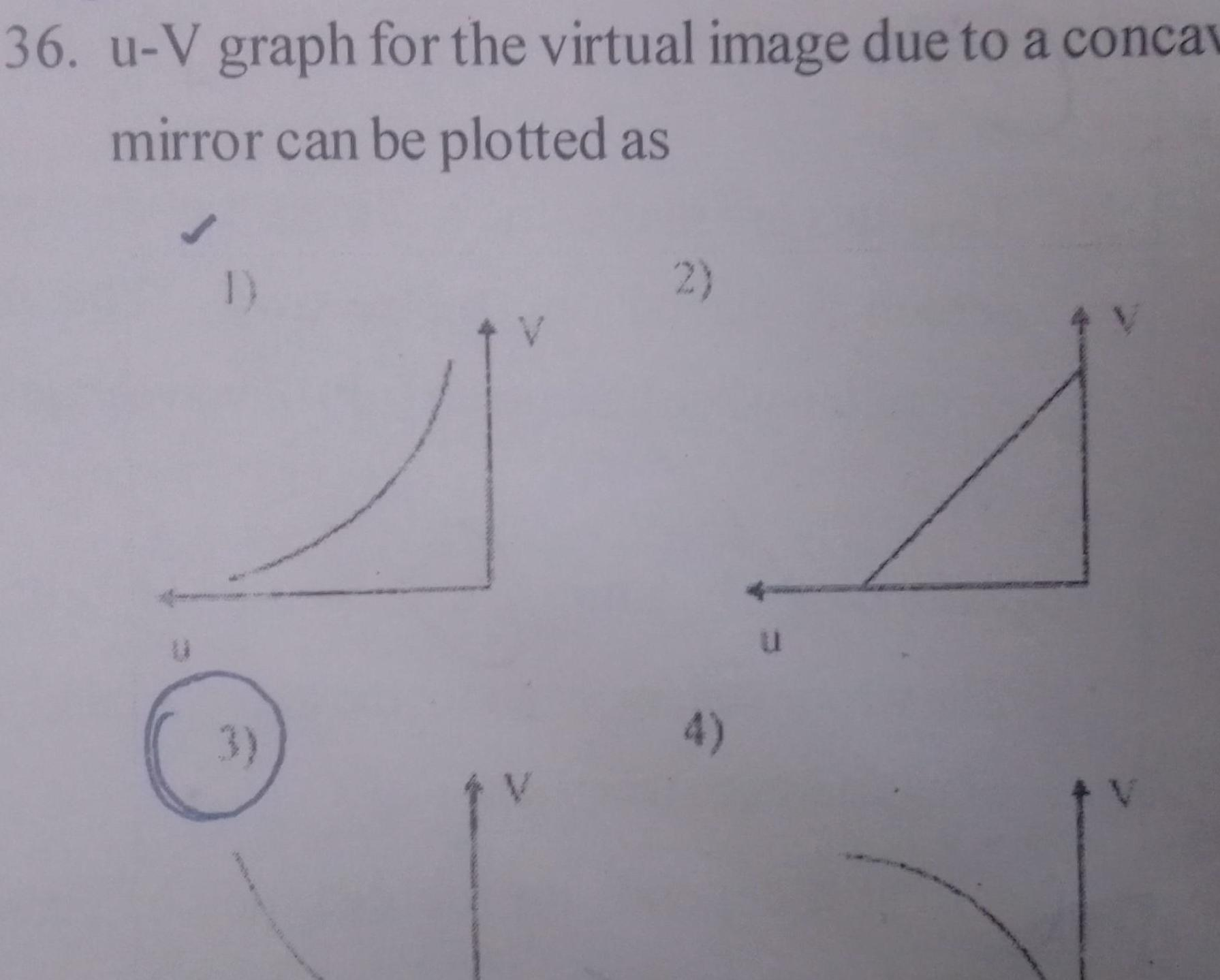Physics
Geometrical Optics
36 u V graph for the virtual image due to a concav mirror can be plotted as 1 3 2 u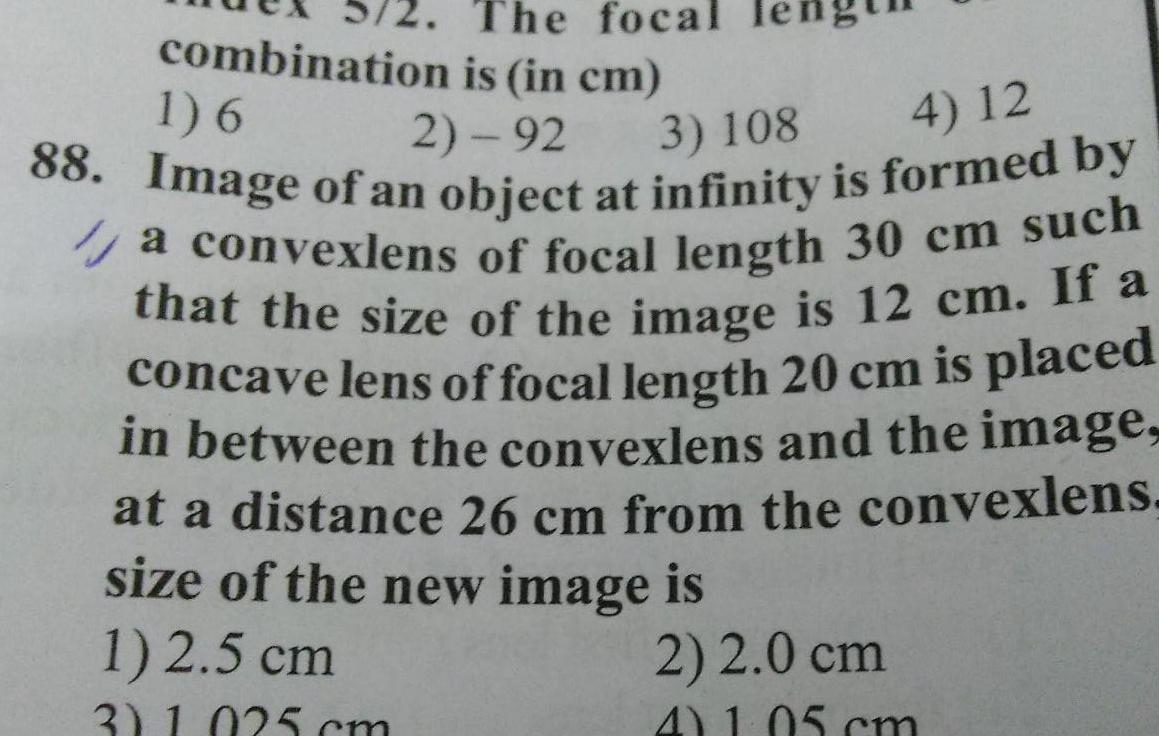Physics
Geometrical Optics
The focal is in cm 2 92 combination 1 6 4 12 88 Image of an object at infinity is formed by 3 108 a convexlens of focal length 30 cm such that the size of the image is 12 cm If a concave lens of focal length 20 cm is placed in between the convexlens and the image at a distance 26 cm from the convexlens size of the new image is 1 2 5 cm 2 2 0 cm 4 1 05 cm 3 1 025 cm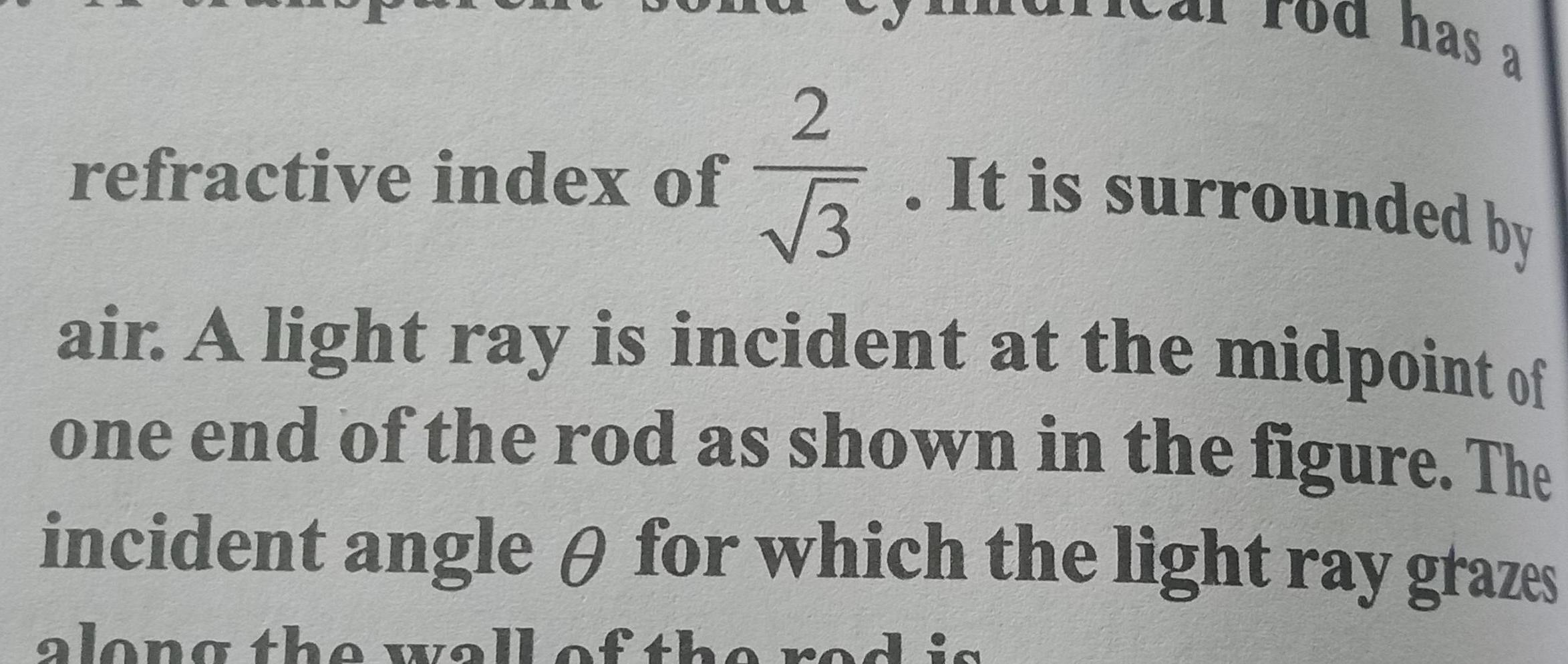Physics
Geometrical Optics
refractive index of 2 3 has a It is surrounded by air A light ray is incident at the midpoint of one end of the rod as shown in the figure The incident angle for which the light ray grazes along the wall of the dis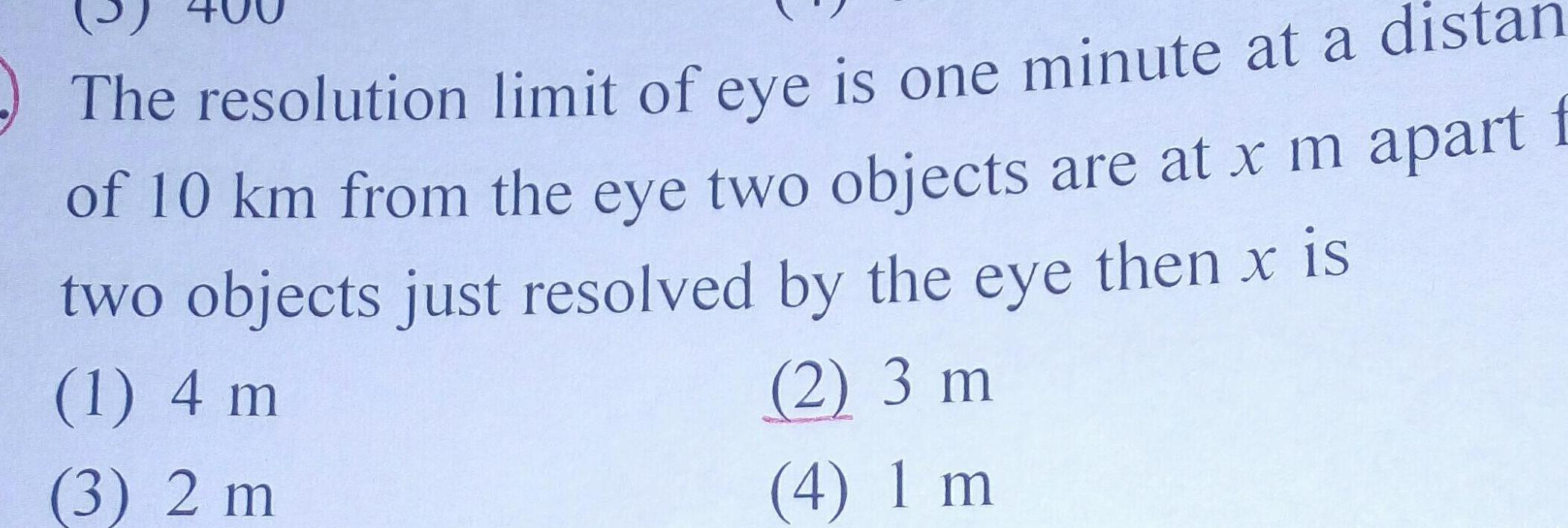Physics
Geometrical Optics
The resolution limit of eye is one minute at a di of 10 km from the eye two objects are at x m apart f two objects just resolved by the eye then x is 1 4 m 2 3 m 3 2 m 4 1 m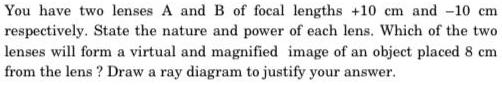Physics
Geometrical Optics
You have two lenses A and B of focal lengths 10 cm and 10 cm respectively State the nature and power of each lens Which of the two lenses will form a virtual and magnified image of an object placed 8 cm from the lens Draw a ray diagram to justify your answer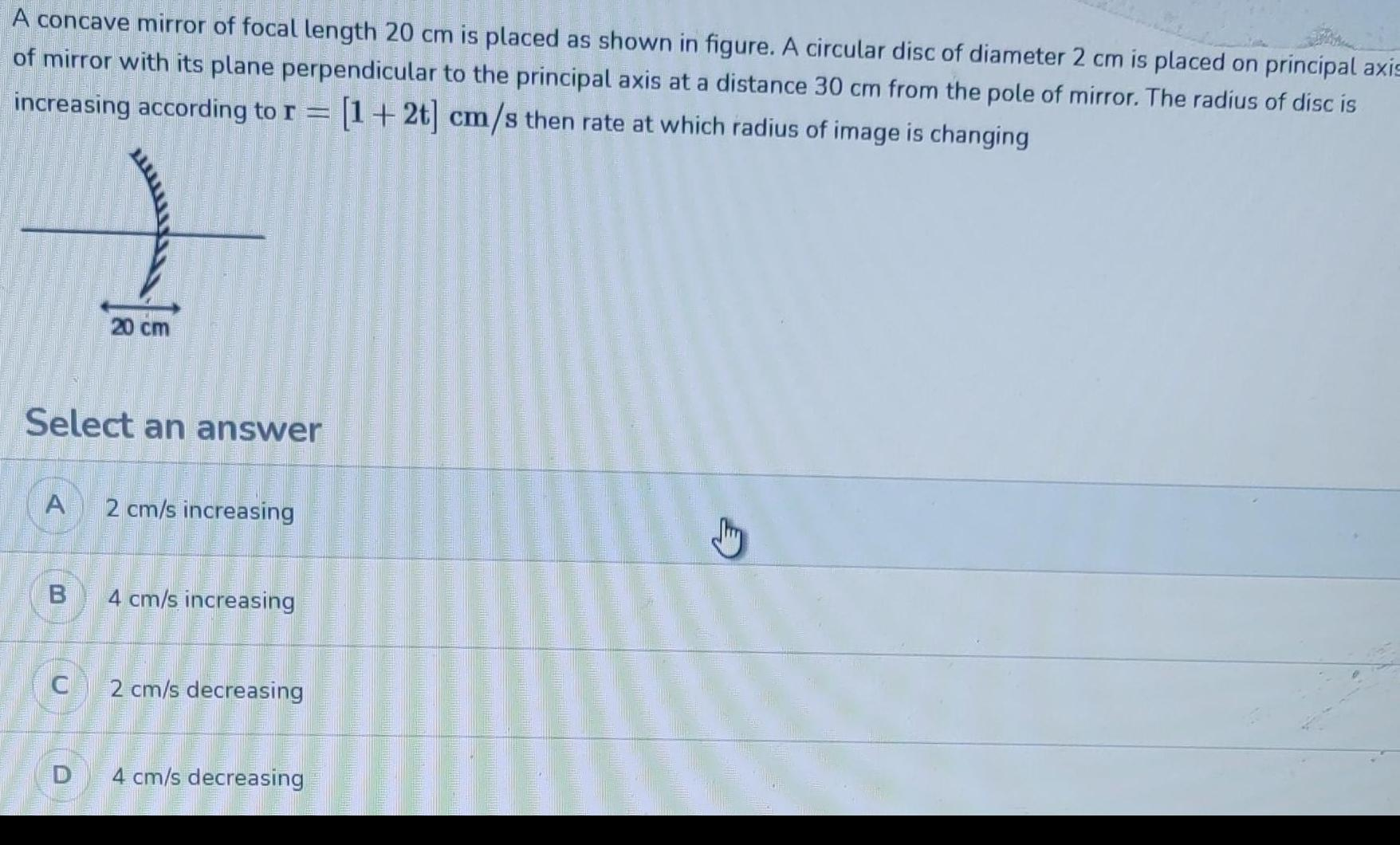Physics
Geometrical Optics
A concave mirror of focal length 20 cm is placed as shown in figure A circular disc of diameter 2 cm is placed on principal axis of mirror with its plane perpendicular to the principal axis at a distance 30 cm from the pole of mirror The radius of disc is increasing according to r 1 2t cm s then rate at which radius of image is changing Select an answer A B C 20 cm D 2 cm s increasing 4 cm s increasing 2 cm s decreasing 4 cm s decreasing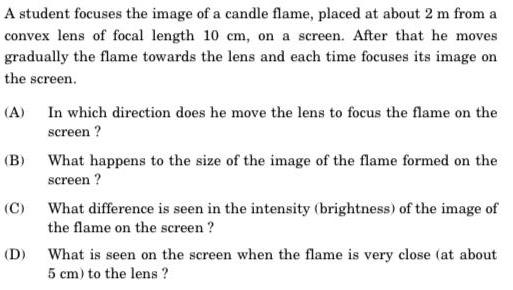Physics
Geometrical Optics
A student focuses the image of a candle flame placed at about 2 m from a convex lens of focal length 10 cm on a screen After that he moves gradually the flame towards the lens and each time focuses its image on the screen A B C In which direction does he move the lens to focus the flame on the screen What happens to the size of the image of the flame formed on the screen What difference is seen in the intensity brightness of the image of the flame on the screen D What is seen on the screen when the flame is very close at about 5 cm to the lens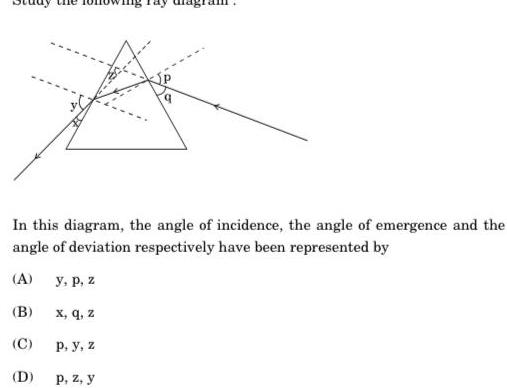Physics
Geometrical Optics
In this diagram the angle of incidence the angle of emergence and the angle of deviation respectively have been represented by A y p z B C D x q z P y z p z y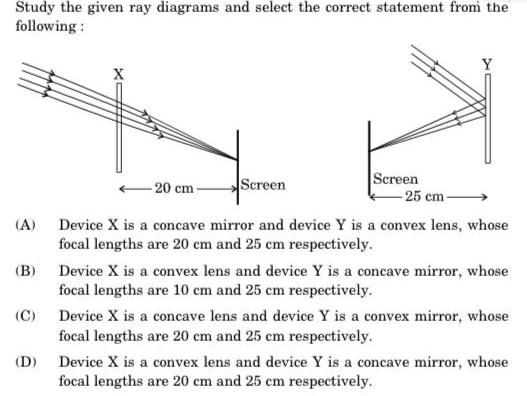Physics
Geometrical Optics
Study the given ray diagrams and select the correct statement from the following A B C D X 20 cm Screen Screen 25 cm Device X is a concave mirror and device Y is a convex lens whose focal lengths are 20 cm and 25 cm respectively Device X is a convex lens and device Y is a concave mirror whose focal lengths are 10 cm and 25 cm respectively Device X is a concave lens and device Y is a convex mirror whose focal lengths are 20 cm and 25 cm respectively Device X is a convex lens and device Y is a concave mirror whose focal lengths are 20 cm and 25 cm respectively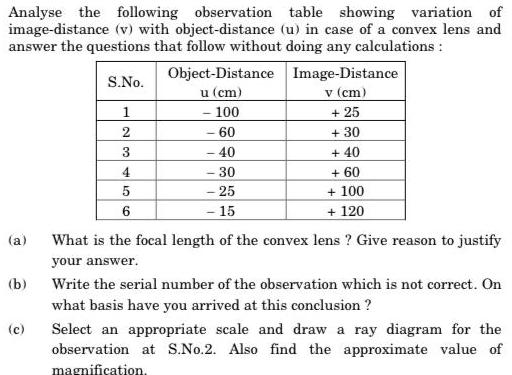Physics
Geometrical Optics
Analyse the following observation table showing variation of image distance v with object distance u in case of a convex lens and answer the questions that follow without doing any calculations a b c S No 1 2 3 4 5 6 Object Distance u cm 100 60 40 30 25 15 Image Distance v cm 25 30 40 60 100 120 What is the focal length of the convex lens Give reason to justify your answer Write the serial number of the observation which is not correct On what basis have you arrived at this conclusion Select an appropriate scale and draw a ray diagram for the observation at S No 2 Also find the approximate value of magnification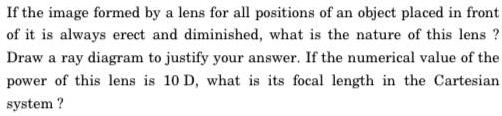Physics
Geometrical Optics
If the image formed by a lens for all positions of an object placed in front of it is always erect and diminished what is the nature of this lens Draw a ray diagram to justify your answer If the numerical value of the power of this lens is 10 D what is its focal length in the Cartesian system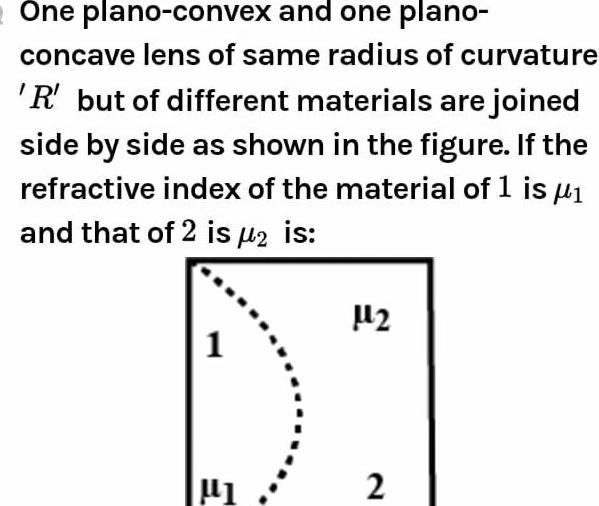Physics
Geometrical Optics
One plano convex and one plano concave lens of same radius of curvature R but of different materials are joined side by side as shown in the figure If the refractive index of the material of 1 is and that of 2 is 2 is 1 1 1 2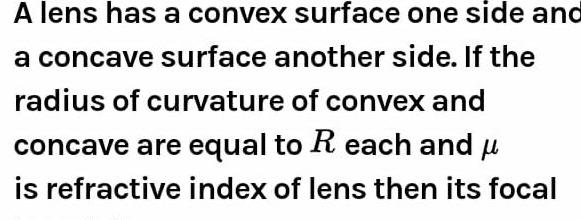Physics
Geometrical Optics
A lens has a convex surface one side and a concave surface another side If the radius of curvature of convex and concave are equal to R each and u is refractive index of lens then its focal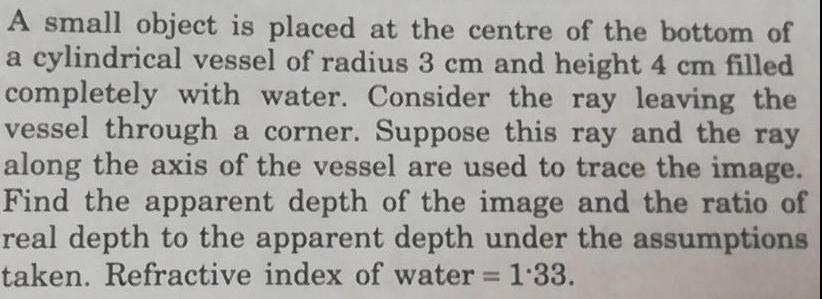Physics
Geometrical Optics
A small object is placed at the centre of the bottom of a cylindrical vessel of radius 3 cm and height 4 cm filled completely with water Consider the ray leaving the vessel through a corner Suppose this ray and the ray along the axis of the vessel are used to trace the image Find the apparent depth of the image and the ratio of real depth to the apparent depth under the assumptions taken Refractive index of water 1 33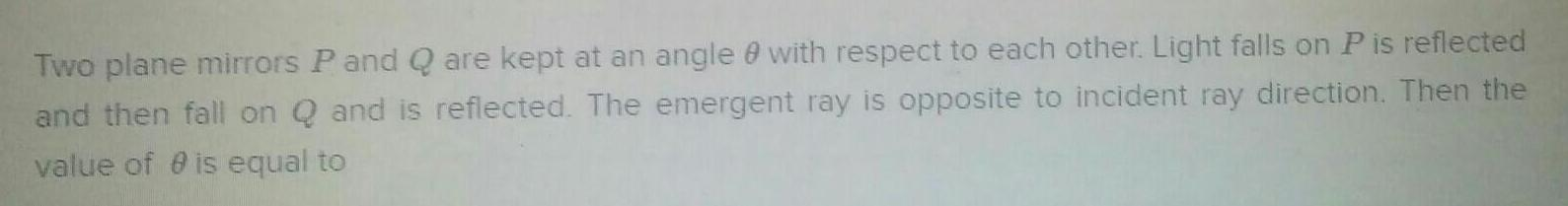Physics
Geometrical Optics
Two plane mirrors P and Q are kept at an angle with respect to each other Light falls on P is reflected and then fall on Q and is reflected The emergent ray is opposite to incident ray direction Then the value of 0 is equal to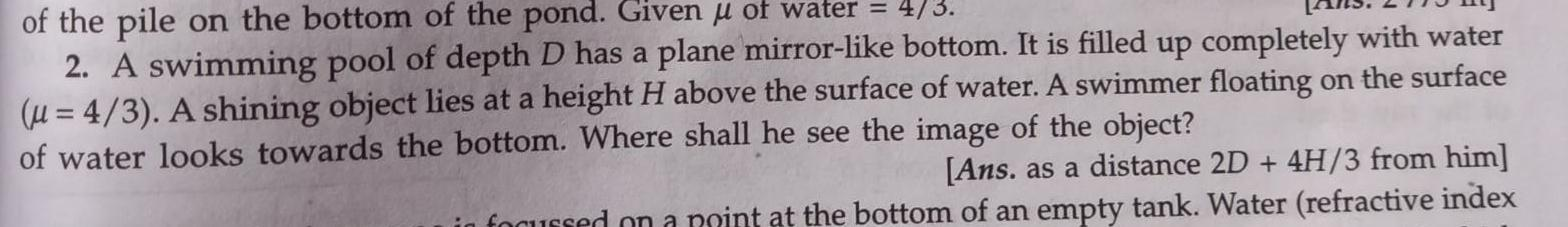Physics
Geometrical Optics
of the pile on the bottom of the pond Given u of water 4 3 2 A swimming pool of depth D has a plane mirror like bottom It is filled up completely with water u 4 3 A shining object lies at a height H above the surface of water A swimmer floating on the surface of water looks towards the bottom Where shall he see the image of the object Ans as a distance 2D 4H 3 from him in focussed on a point at the bottom of an empty tank Water refractive index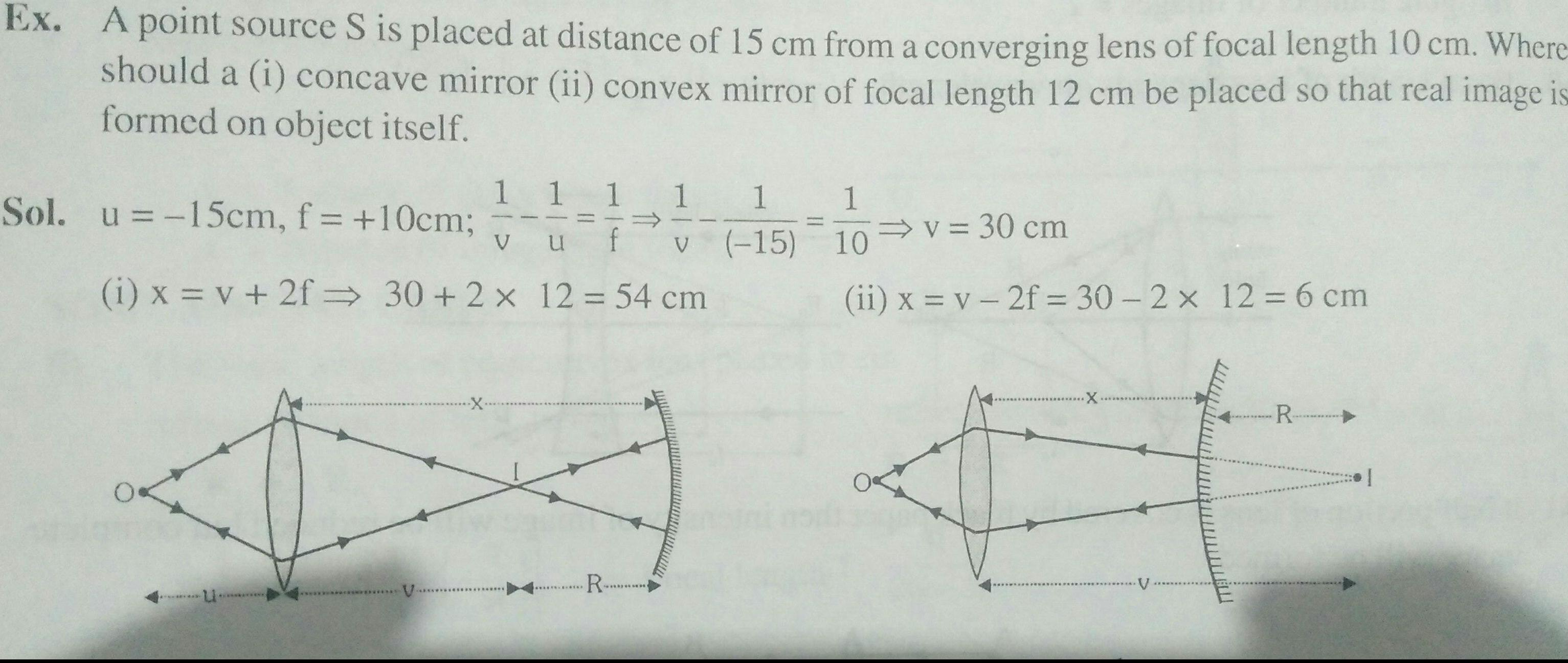Physics
Geometrical Optics
Ex A point source S is placed at distance of 15 cm from a converging lens of focal length 10 cm Where should a i concave mirror ii convex mirror of focal length 12 cm be placed so that real image is formed on object itself 1 1 1 1 V u v i x v 2f 30 2 x 12 54 cm Sol u 15cm f 10cm R 1 15 1 10 v 30 cm ii x v 2f 30 2 x 12 6 cm X V R I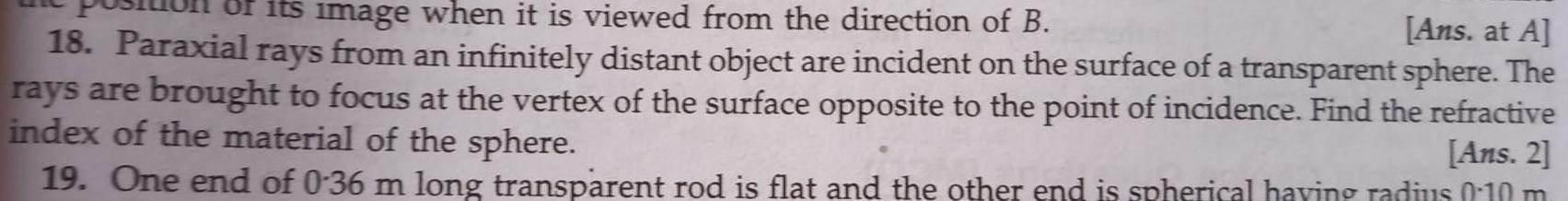Physics
Geometrical Optics
of its image when it is viewed from the direction of B Ans at A 18 Paraxial rays from an infinitely distant object are incident on the surface of a transparent sphere The rays are brought to focus at the vertex of the surface opposite to the point of incidence Find the refractive index of the material of the sphere Ans 2 19 One end of 0 36 m long transparent rod is flat and the other end is spherical having radius 0 10 m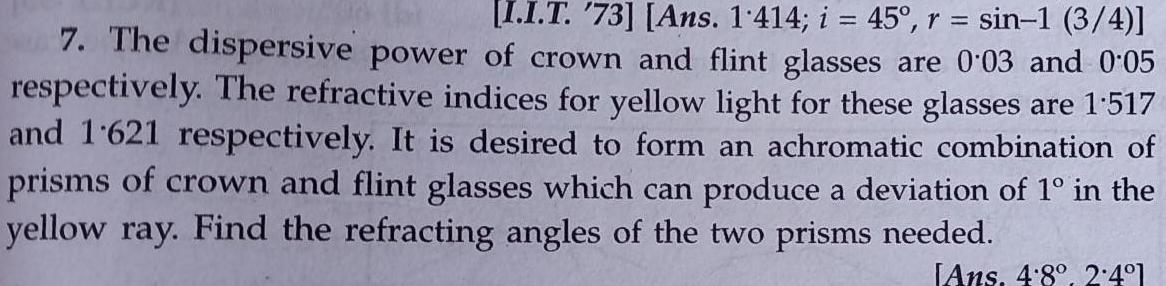Physics
Geometrical Optics
I I T 73 Ans 1 414 i 45 r sin 1 3 4 7 The dispersive power of crown and flint glasses are 0 03 and 0 05 respectively The refractive indices for yellow light for these glasses are 1 517 and 1621 respectively It is desired to form an achromatic combination of prisms of crown and flint glasses which can produce a deviation of 1 in the yellow ray Find the refracting angles of the two prisms needed Ans 4 8 2 4 1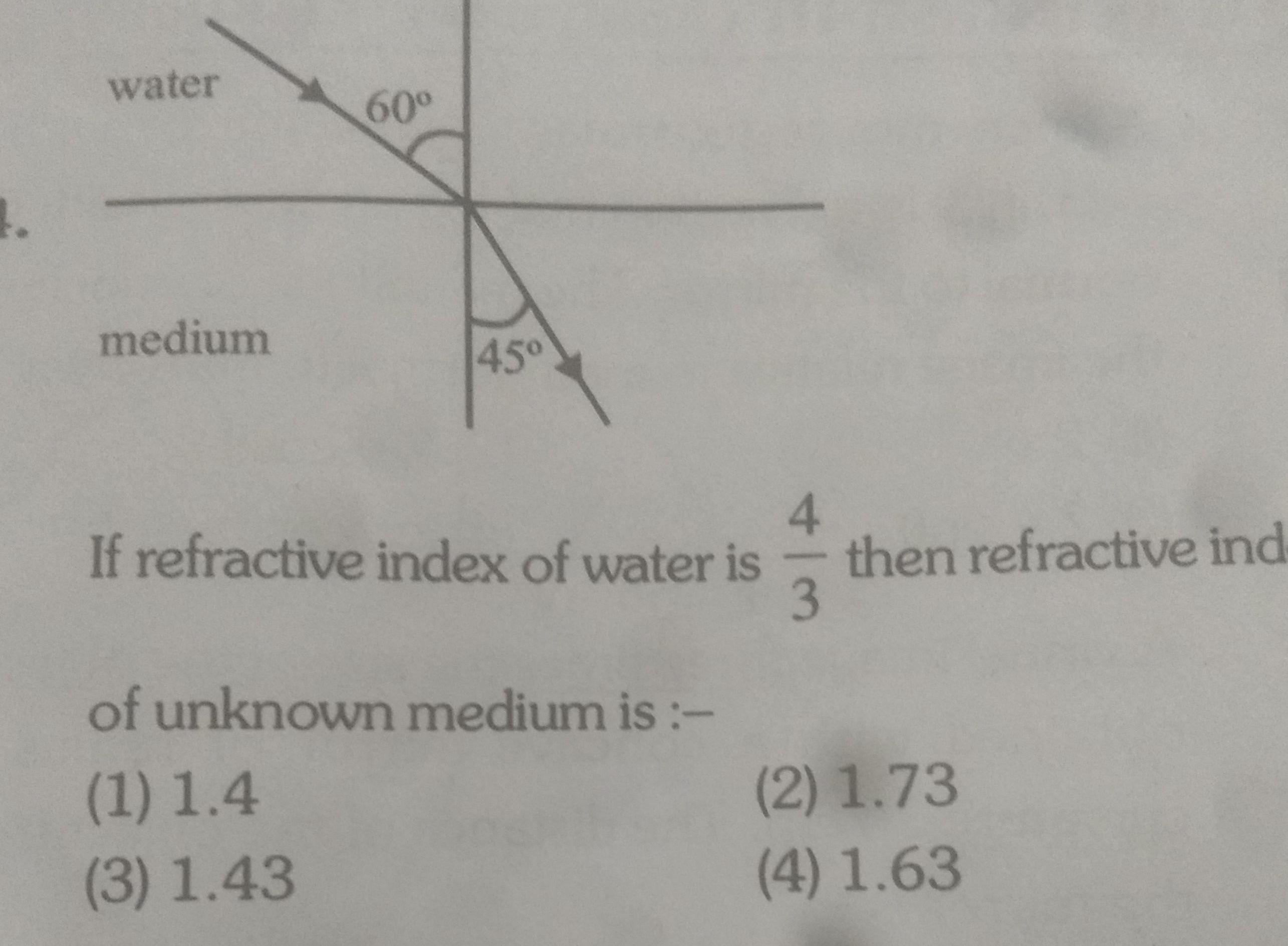Physics
Geometrical Optics
1 water medium 60 45 If refractive index of water is 3 of unknown medium is 1 1 4 3 1 43 then refractive ind 2 1 73 4 1 63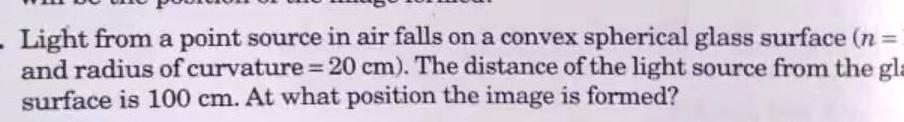Physics
Geometrical Optics
Light from a point source in air falls on a convex spherical glass surface n and radius of curvature 20 cm The distance of the light source from the gla surface is 100 cm At what position the image is formed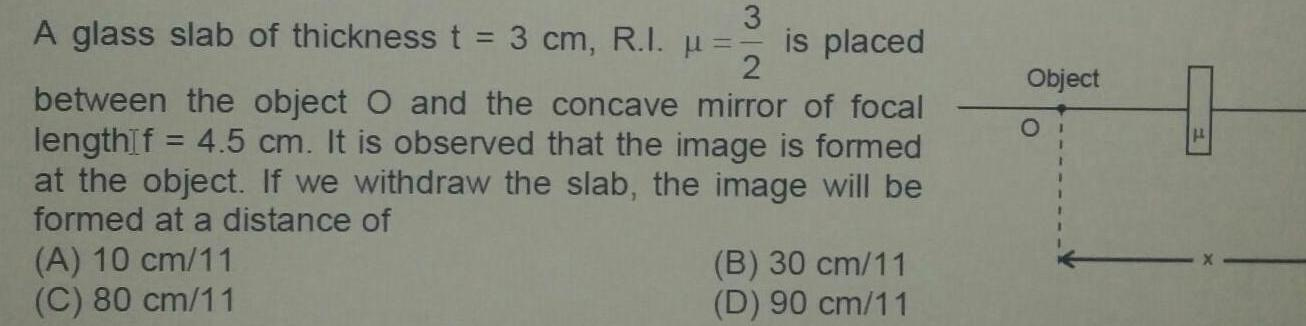Physics
Geometrical Optics
3 A glass slab of thickness t 3 cm R I 2 between the object O and the concave mirror of focal lengthIf 4 5 cm It is observed that the image is formed at the object If we withdraw the slab the image will be formed at a distance of A 10 cm 11 C 80 cm 11 is placed B 30 cm 11 D 90 cm 11 Object F